# Operations On Rational Numbers

Rational Numbers are any real numbers that can be expressed as a simple fraction or ratio. Thus, a rational number can be defined as any number which can be written in the form where p and q are any two integers and q not equal to zero (q ≠ 0). Here p and q are the numerator and denominator of a rational number respectively and denominator can never be zero. Ex:8/5, 3, 2/900  Any number i.e. whole numbers, natural numbers, integers, decimal numbers and fractions can be expressed in this form of numerator/ denominator. A set of rational number is denoted by the letter Q. Like all other real numbers arithmetic operations are applicable to rational numbers as well. Arithmetical operations on rational numbers include addition, subtraction, multiplication and division.

Rational numbers can be two types they are numbers with same denominators and numbers with different denominators. The procedures to evaluate both these types of numbers are different. Arithmetical operations on rational numbers with same denominators are much easier task. For any operations on rational numbers with same denominators we can simply operate it by keeping a common denominator. Let’s learn various operations on rational numbers with examples.

Addition of rational numbers with same denominators: Consider two rational numbers 2/9 and 3/9, here both have different numerators but same denominators. To add these, we add both the numerators keeping common denominator.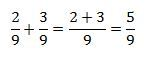Addition of rational numbers with different denominators:

To add rational numbers with different denominators convert them to rational numbers with same denominators and then add.

In order to convert rational numbers to numbers with same denominators take the LCM of the denominators. Then multiply both rational numbers by this LCM and we will get equivalent rational numbers with this LCM as the denominator.

Example 1: Add 7/6 and 2/9

Solution: To evaluate7/6+2/9

• Convert this to rational numbers with same denominators.
• LCM of 6 and 9 is 18
• Multiply 18 by both rational numbers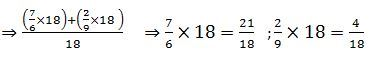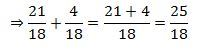Additive Inverse Opposite of a number is the number with same magnitude of opposite sign. -1 is the opposite of 1. The sum of a number and its opposite is always zero.  This is same for rational numbers also. Two rational numbers are additive inverse of each other when their sum is zero. Thus, all opposite numbers are additive inverse to each other.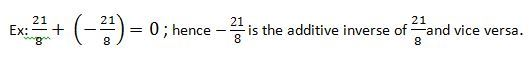### Subtraction of rational number

Subtraction of rational numbers with same and/or different denominators can be done like addition. Subtraction of rational numbers will be easy by transforming subtraction problem into addition problem. This can be done in two steps;

• Change the subtraction sign (-) into addition sign (+)
• Take the additive inverse of the rational number come after the sign.

Simply, take the additive inverse of the number after the sign and add them.

Example 2: Subtract  5/2 and -4/7

Solution: We have to subtract 5/2 and -4/7

For this, first convert subtraction problem into addition problem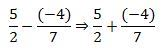Take  the additive inverse of the number after the sign. Here, -4/7  is the number after the sign.

Here, LCM of 2 and 7 are 14.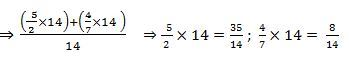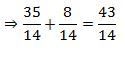### Multiplication of rational number

Multiplication of rational numbers is as simple as multiplication of integers. In general, product of two rational numbers will equal to the product of the numerators divided by the product of the denominators.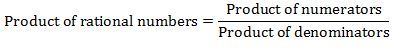To find the product of a rational number and an integer, keep the denominator unchanged and multiply the numerators. To find the product of rational numbers, multiply the numerators and multiply denominators and write in the form given above.

Example 3: Multiply 3/8 and -9/11

Solution: To find the product, multiply the numerators and multiply denominators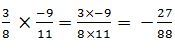Example 4: Divide 11/12 and 7

Solution:To find the product, multiply the numerators and keep the denominator unchanged.

We know, 7=1/7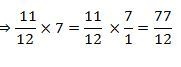Division of rational number

Divisions of rational numbers are similar to the divisions of fractions. To find the quotient of rational numbers, we need to recollect the concept of reciprocal of a rational number. Reciprocal of a rational number is nothing but the swapping of the numerator and denominator of that rational number i.e. invert the given the rational number will give you the reciprocal of that rational number. For example, 7/6 is the reciprocal of 6/7 . The product of a number and its reciprocal will always be 1.

In order to divide rational number, first change the division sign  to . Then take the reciprocal of the number after the sign and multiply.

Example 5: Divide 11/12 and  7/4

Solution: To evaluate 11/12÷7/4

• Change the division sign ÷ to x.
• Take the reciprocal of 7/4 which is equal to 4/7.
• Multiply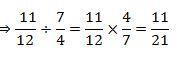To solve more problems on the topic, download Byju’s – The Learning App from Google Play Store and watch interactive videos. Also, take free tests to practice for exams.